International
Tables for
Crystallography
Volume C
Mathematical, physical and chemical tables
Edited by E. Prince

International Tables for Crystallography (2006). Vol. C, ch. 8.4, pp. 704-705

## Section 8.4.3. Comparison of different models

E. Princea and C. H. Spiegelmanb

aNIST Center for Neutron Research, National Institute of Standards and Technology, Gaithersburg, MD 20899, USA, and bDepartment of Statistics, Texas A&M University, College Station, TX 77843, USA

### 8.4.3. Comparison of different models

| top | pdf |

Tests based on F or the R ratio have several limitations. One important one is that they are applicable only when the parameters of one model form a subset of the parameters of the other. Also, the F test makes no distinction between improvement in fit as a result of small improvements throughout the entire data set and a large improvement in a small number of critically sensitive data points. A test that can be used for comparing arbitrary pairs of models, and that focuses attention on those data points that are most sensitive to differences in the models, was introduced by Williams & Kloot (1953; also Himmelblau, 1970; Prince, 1982).

Consider a set of observations,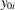, and two models that predict values for these observations,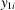and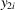, respectively. We determine the slope of the regression line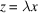, where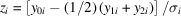, and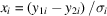. Suppose model 1 is a perfect fit to the data, which have been measured with great precision, so that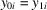for all i. Under these conditions, λ = +1/2. Similarly, if model 2 is a perfect fit, λ = −1/2. Real experimental data, of course, are subject to random error, and |λ| in general would be expected to be less than 1/2. A least-squares estimate of λ is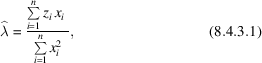and it has an estimated variance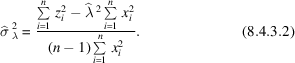The hypothesis that the two models give equally good fits to the data can be tested by considering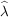to be an unconstrained, one-parameter fit that is to be compared with a constrained, zero-parameter fit for which λ = 0. A p.d.f. for making this comparison can be derived from an F distribution with ν1 = 1 and ν2 = ν = (n − 1).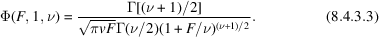If we let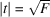, and use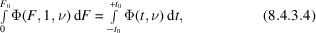we can derive a p.d.f. for t, which is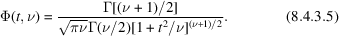This p.d.f. is known as Student's t distribution with ν degrees of freedom. Setting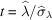, the c.d.f. Ψ(t, ν) can be used to test the alternative hypotheses λ = 0 and λ = ±1/2. Table 8.4.3.1gives the values of t for which the c.d.f. Ψ(t, ν) has various values for various values of ν. Fortran code for the program from which this table was generated appears in Prince (1994).

 Table 8.4.3.1| top | pdf | Values of t for which the c.d.f. Ψ(t, ν) has the values given in the column headings, for various values of ν
ν0.750.900.950.990.995
1 1.0000 3.0777 6.3138 31.8206 63.6570
2 0.8165 1.8856 2.9200 6.9646 9.9249
3 0.7649 1.6377 2.3534 4.5407 5.8409
4 0.7407 1.5332 2.1319 3.7469 4.6041
6 0.7176 1.4398 1.9432 3.1427 3.7074
8 0.7064 1.3968 1.8596 2.8965 3.3554
10 0.6998 1.3722 1.8125 2.7638 3.1693
12 0.6955 1.3562 1.7823 2.6810 3.0546
14 0.6924 1.3450 1.7613 2.6245 2.9769
16 0.6901 1.3368 1.7459 2.5835 2.9208
20 0.6870 1.3253 1.7247 2.5280 2.8453
25 0.6844 1.3164 1.7081 2.4851 2.7874
30 0.6828 1.3104 1.6973 2.4573 2.7500
35 0.6816 1.3062 1.6896 2.4377 2.7238
40 0.6807 1.3031 1.6839 2.4233 2.7045
50 0.6794 1.2987 1.6759 2.4033 2.6778
60 0.6786 1.2958 1.6707 2.3901 2.6603
80 0.6776 1.2922 1.6641 2.3739 2.6387
100 0.6770 1.2901 1.6602 2.3642 2.6259
120 0.6765 1.2886 1.6577 2.3578 2.6174

Again, it must be understood that the results of these statistical comparisons do not imply that either model is a correct one. A statistical indication of a good fit says only that, given the model, the experimenter should not be surprised at having observed the data values that were observed. It says nothing about whether the model is plausible in terms of compatibility with the laws of physics and chemistry. Nor does it rule out the existence of other models that describe the data as well as or better than any of the models tested.

### References

Himmelblau, D. M. (1970). Process analysis by statistical methods. New York: John Wiley.
Prince, E. (1982). Comparison of the fits of two models to the same data set. Acta Cryst. B38, 1099–1100.
Prince, E. (1994). Mathematical techniques in crystallography and materials science, 2nd ed. Berlin/Heidelberg/New York/London/Paris/Tokyo/Hong Kong/Barcelona/Budapest: Springer-Verlag.
Williams, E. J. & Kloot, N. H. (1953). Interpolation in a series of correlated observations. Aust. J. Appl. Sci. 4, 1–17.